2021 NFL Comeback Player of the Year Odds
-300
0.33 to 1Dak Prescott
51.9% implied probability

+800
8 to 1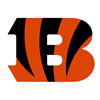Joe Burrow
7.7% implied probability

+1600
16 to 1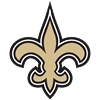Jameis Winston
4.1% implied probability

+1800
18 to 1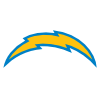Derwin James
3.6% implied probability

+2000
20 to 1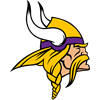Danielle Hunter
3.3% implied probability

+2500
25 to 1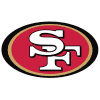Nick Bosa
2.7% implied probability

+2500
25 to 1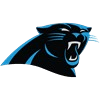Sam Darnold
2.7% implied probability

+3000
30 to 1Christian McCaffrey
2.2% implied probability

+4000
40 to 1O.J. Howard
1.7% implied probability

+4000
40 to 1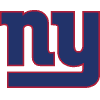Saquon Barkley
1.7% implied probability

+5000
50 to 1Anthony Barr
1.4% implied probability

+5000
50 to 1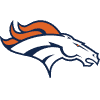Courtland Sutton
1.4% implied probability

+5000
50 to 1George Kittle
1.4% implied probability

+5000
50 to 1James Conner
1.4% implied probability

+5000
50 to 1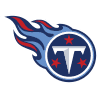Julio Jones
1.4% implied probability

+5000
50 to 1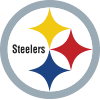Melvin Ingram
1.4% implied probability

+5000
50 to 1Richard Sherman
1.4% implied probability

+5000
50 to 1Von Miller
1.4% implied probability

+5000
50 to 1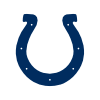Carson Wentz
1.4% implied probability

+5000
50 to 1Jimmy Garoppolo
1.4% implied probability

+5000
50 to 1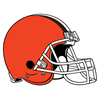Odell Beckham
1.4% implied probability

+5000
50 to 1Devin Bush
1.4% implied probability

+6000
60 to 1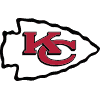Laurent Duvernay-Tardif
1.1% implied probability

+6000
60 to 1Leighton Vander Esch
1.1% implied probability